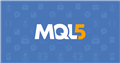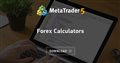What can i use instead of SYMBOL_TRADE_TICK_VALUE ? I am calculating lot size based on risk percentage. Its giving me right lots on EURUSD,GBPUSD but it gives wrong valuein US30, USDJPY. Can anyone help me. I am calculating lot size based on risk percentage. Its giving me right l
```double calcLots(double riskPercent,double slPoints){

ticksize = NormalizeDouble(ticksize,_Digits);

tickvalue = NormalizeDouble(tickvalue,_Digits);

double lotstep = SymbolInfoDouble(_Symbol,SYMBOL_VOLUME_STEP);
lotstep = NormalizeDouble(lotstep,_Digits);

Print("tick size::",ticksize,"...tick value::",tickvalue,"...lot step ::",lotstep,"...sl Points::",slPoints);

if(ticksize == 0 || tickvalue == 0 || lotstep == 0){
Print(__FUNCTION__,">Lotsize cannot be calculated..");
return 0;

}

double risk = AccountInfoDouble(ACCOUNT_BALANCE) * riskPercent / 100;
double moneyPerLotstep = (slPoints / ticksize) * tickvalue * lotstep;
moneyPerLotstep = NormalizeDouble(moneyPerLotstep,_Digits);

Print("risk amount for the trade is..",risk," and monry per lot step ==",moneyPerLotstep);

if(moneyPerLotstep == 0){
Print(__FUNCTION__,">Lot size cannot be calculated>.");
return 0;
}

double lots = MathFloor(risk / moneyPerLotstep) * lotstep;
Print("Lots calculated is..",lots);
lots = NormalizeDouble(lots,_Digits);
lots = (int)(lots/SymbolInfoDouble(_Symbol,SYMBOL_VOLUME_STEP))* SymbolInfoDouble(_Symbol,SYMBOL_VOLUME_STEP);
lots = MathMin(lots,SymbolInfoDouble(_Symbol,SYMBOL_VOLUME_MAX));
lots = MathMax(lots,SymbolInfoDouble(_Symbol,SYMBOL_VOLUME_MIN));

return lots;

}
```

ots on EURUSD,GBPUSD but it gives wrong valuein US30, USDJPY. Can anyone help me.

Abhishek Yadav: What can i use instead of SYMBOL_TRADE_TICK_VALUE ? I am calculating lot size based on risk percentage. Its giving me right lots on EURUSD,GBPUSD but it gives wrong valuein US30, USDJPY. Can anyone help me. I am calculating lot size based on risk percentage. Its giving me right lots on EURUSD,GBPUSD but it gives wrong valuein US30, USDJPY. Can anyone help me.
1. What are the values in the log for US30 and USDJPY for this code?
`Print("tick size::",ticksize,"...tick value::",tickvalue,"...lot step ::",lotstep,"...sl Points::",slPoints);`
2. If your stop-loss is in points then first convert it into tick size units or use the point value instead. Don't mix "point size" and "tick size" (see below).

3. In MQL5 use the OrderCalcProfit function instead so that it can adapt to the different symbol profit calculation types (see below).

Symbol Point Value

Fernando Carreiro, 2022.05.18 21:05

```double
dbTickSize   = SymbolInfoDouble( _symbol, SYMBOL_TRADE_TICK_SIZE  ), // Tick size
dbTickValue  = SymbolInfoDouble( _symbol, SYMBOL_TRADE_TICK_VALUE ), // Tick value
dbPointSize  = SymbolInfoDouble( _symbol, SYMBOL_POINT ),            // Point size
dbPointValue = dbTickValue * dbPointSize / dbTickSize;               // Point value
```
Remember, it's best to use tick size and tick value in your calculations, instead of point size and its value.

Tick size vs Point(), can be a little tricky in Multicurrency EA

Fernando Carreiro, 2022.03.09 12:11

Tick Size and Point Size can be very different especially on stocks and other symbols besides forex.

Always use Tick Size to adjust and align your prices, not the point size. In essence, make sure that your price quotes, are properly aligned to the Tick size (see following examples).

```...
double tickSize = SymbolInfoDouble( _Symbol, SYMBOL_TRADE_TICK_SIZE );
...
double normalised_price = round( price / tick_size ) * tick_size;
...
// Or use a function
double Round2Ticksize( double price )
{
double tick_size = SymbolInfoDouble( _Symbol, SYMBOL_TRADE_TICK_SIZE );
return( round( price / tick_size ) * tick_size );
};```

Fernando Carreiro, 2022.08.23 17:41

You can! These are the steps I take. I supply the function with a lot size equal to the “Max Lot Size” allowed for the symbol in question, then calculate the ratio needed to achieve the fractional risk that I wish to apply, to get the correct volume for the order. I then align that with the “Lot Step” and finally check it against both the maximum and minimum allowed lots for the symbol.

The reason I use the “maximum” lots instead of just “1.0” lots as a reference value is because there is no guarantee that the value of 1.0 is within the minimum and maximum values allowed. Given that using 1.0, or the maximum, gives equivalent results anyway (by using the ratio method), I choose to use the “max lots” as the reference point which also offers the most precision for the calculation.

Something like this ...

```// This code will not compile. It is only a example reference

if( OrderCalcProfit( eOrderType, _Symbol, dbLotsMax, dbPriceOpen, dbPriceStopLoss, dbProfit ) )
{
dbOrderLots = fmin( fmax( round( dbRiskMax * dbLotsMax / ( -dbProfit * dbLotsStep ) )
* dbLotsStep, dbLotsMin ), dbLotsMax );

// the rest of the code ...
};```

Why am I limited to under 1 in the Lots input.

Fernando Carreiro, 2023.01.05 20:50

There could be a bug in your code, but you should also check the contract specifications of the symbol being traded.

```// Variables for Symbol Volume Conditions
double
dblLotsMinimum = SymbolInfoDouble( _Symbol, SYMBOL_VOLUME_MIN  ),
dblLotsMaximum = SymbolInfoDouble( _Symbol, SYMBOL_VOLUME_MAX  ),
dblLotsStep    = SymbolInfoDouble( _Symbol, SYMBOL_VOLUME_STEP );

// Adjust Volume for allowable conditions
double
dblLotsAdjusted = fmin(  dblLotsMaximum,                             // Prevent too greater volume
fmax(  dblLotsMinimum,                             // Prevent too smaller volume
round( dblLots / dblLotsStep ) * dblLotsStep ) );  // Align to Step value```Documentation on MQL5: Trade Functions / OrderCalcProfit
• www.mql5.com

Fernando Carreiro #:
1. What are the values in the log for US30 and USDJPY for this code:
2. If your stop-loss is in points then first convert it into tick size units or use the point value instead. Don't mix "point size" and "tick size" (see below).

3. In MQL5 use the OrderCalcProfit function instead so that it can adapt to the different symbol profit calculation types (see below).

Hey Thnks. Everything works fine in live market but when i try to test it , it gives me wrong lot.

Abhishek Yadav #: Hey Thnks. Everything works fine in live market but when i try to test it , it gives me wrong lot.

You did not answer my question?

Consider applying what I have described, because your calculations and code are incorrect!

Maybe you have some specific tester settings that are causing the problem – check the symbol properties in tester and see if the values are correct. It should be working fine if the real market is calculating properly.

And it would be helpful if you show us the values you think are wrong and why they’re wrong – we don’t know what you really mean by “wrong values”

Fernando Carreiro #:

You did not answer my question?

Consider applying what I have described, because your calculations and code are incorrect!

For USDJPY tick size = 0.001, tick value is 100, lot step = 0.01. I cant see the tick size and tick value in my broker's symbols properties. I found tick size of USDJPY as 0.005 which is wrong in my case . kindly help me. This problem shows in US30 and which does not have USD as currency. Thank You.

Marzena Maria Szmit #:

Maybe you have some specific tester settings that are causing the problem – check the symbol properties in tester and see if the values are correct. It should be working fine if the real market is calculating properly.

And it would be helpful if you show us the values you think are wrong and why they’re wrong – we don’t know what you really mean by “wrong values”

For USDJPY tick size = 0.001, tick value is 100, lot step = 0.01. I cant see the tick size and tick value in my broker's symbols properties. I found tick size of USDJPY as 0.005 which is wrong in my case . kindly help me. This problem shows in US30 and which does not have USD as currency. Thank You.

Fernando Carreiro #:

You did not answer my question?

Consider applying what I have described, because your calculations and code are incorrect!

And for US30 tick size =  0.01, tick value =  0.01, lot step =0.1 . where as broker shows bots tick size and tick value 0. Frustated"" Can anyone help me.